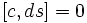# Derivation-invariance is centralizer-closed

This article gives the statement, and possibly proof, of a Lie subring property (i.e., derivation-invariant subring) satisfying a Lie subring metaproperty (i.e., centralizer-closed Lie subring property)
View all Lie subring metaproperty satisfactions | View all Lie subring metaproperty dissatisfactions |Get help on looking up metaproperty (dis)satisfactions for Lie subring properties
Get more facts about derivation-invariant subring |Get facts that use property satisfaction of derivation-invariant subring | Get facts that use property satisfaction of derivation-invariant subring|Get more facts about centralizer-closed Lie subring property
ANALOGY: This is an analogue in Lie rings of a fact encountered in group. The old fact is: characteristicity is centralizer-closed.
Another analogue to the same fact, in the same new context, is: characteristicity is centralizer-closed for Lie rings
View other analogues of characteristicity is centralizer-closed|View other analogues from group to Lie ring (OR, View as a tabulated list)

## Statement

### Verbal statement

The centralizer of a derivation-invariant subring of a Lie ring is also derivation-invariant.

## Proof

Given: A Lie ring$L$, a derivation-invariant subring$S$ of$L$.$C = C_L(S)$ is the set of$a \in L$ such that$[a,s] = 0$ for all$s \in S$.

To prove:$C$ is also derivation-invariant, i.e.,$d(C) \subseteq C$ for any$d \in \operatorname{Der}(L)$.

Proof: Suppose$d \in \operatorname{Der}(L)$. For any$c \in C$ and$s \in S$, we need to show that$[dc,s] = 0$.

For this, note that, by the Leibniz rule property of derivations:$d([c,s]) = [dc,s] + [c,ds]$.

Since$[c,s] = 0$, the left side is zero. Further, since$S$ is derivation-invariant,$ds \in S$, so$[c,ds] = 0$. This gives$[dc,s] = 0$ as required.